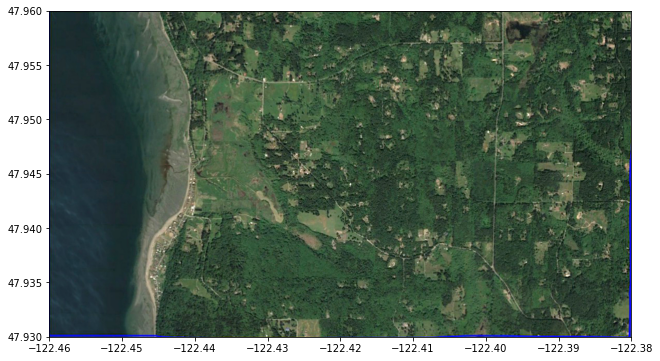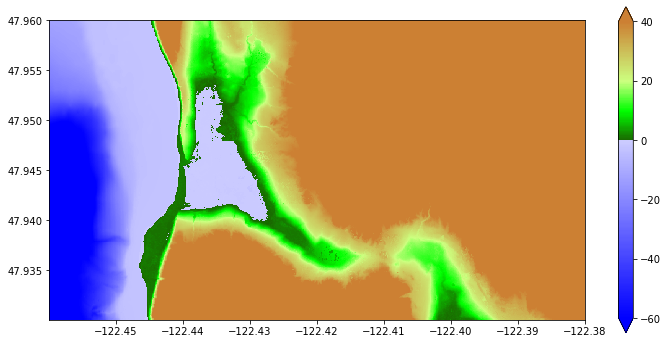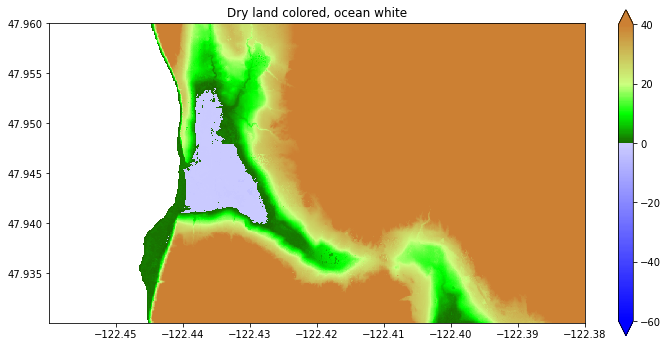# Force Cells to be Dry Initially¶

This Jupyter notebook can be found in this collection of Clawpack apps as the file \$CLAW/apps/notebooks/geoclaw/ForceDry.ipynb. To run this notebook, install Clawpack, and clone the apps repository. A static view of this and other notebooks can be found in the Clawpack Gallery of Jupyter notebooks.

The MarchingFront.ipynb notebook illustrated tools to select points from a topography DEM that satisfy given constraints on elevation, and how this can be used to determine dry land behind dikes.

In this notebook we explore this more and show how to define an array that can be read into GeoClaw and used when initializing the water depth during the creation of new grid patches.

See also the documentation page Force Cells to be Dry Initially.

We define an rectangular array force_dry_init that is aligned with cell centers of the computational grid at some resolution (typically the finest resolution) and that has the value force_dry_init[i,j] = 1 to indicate cells that should be initialized to dry (h[i,j] = 0) regardless of the value of the GeoClaw topography B[i,j] in this cell. If force_dry_init[i,j] = 0 the the cell is initialized in the usual manner, which generally means

h[i,j] = max(0, sea_level - B[i,j]).

Notes:

• Another new feature allows initializing the depth so that the surface elevation eta is spatially varying rather than using a single scalar value sea_level everywhere. That feature is described in VariableEtaInit.ipynb. If used in conjunction with a force_dry_init array, force_dry_init[i,j] = 1 still indicates that the cell should be dry while elsewhere the "usual" thing is done.

• In the project archived at IslandWhidbeyTHA_2019 project webpage, rather than a force_dry_init array, the masking array was called allow_wet_init with the meaning of 0 and 1 reversed. However, it seems more sensible to have the default be the "usual" initialization since the force_dry_init array may only cover a subset of the computational domain where the special initialization is required.

• The current implementation allows only one force_dry_init array but ideally this would be generalized to allow multiple arrays covering different subsets of the domain, and maybe at different grid resolutions?

• Typically the force_dry_init array is computed from a DEM file at the desired resolution, using the marching front algorithm defined in MarchingFront.ipynb. But recall that the GeoClaw topography value B[i,j] does not agree with the DEM value Z[i,j] even if the cell center is aligned with the DEM point due to the way B is computed by averaging over piecewise bilinear functions that interpolate the Z values. So one has to be careful not to set force_dry_init[i,j] = 1 in a cell close to the shore simply because Z > 0 at this point since the B value might be negative in the cell. This is dealt with in the examples below by doing some buffering.

## Examples¶

First import some needed modules and set up color maps.

In :
%matplotlib inline

In :
from pylab import *
import os,sys
from numpy import ma # masked arrays
from clawpack.visclaw import colormaps, plottools
from clawpack.amrclaw import region_tools
from clawpack.geoclaw import topotools, marching_front

In :
zmin = -60.
zmax = 40.

land_cmap = colormaps.make_colormap({ 0.0:[0.1,0.4,0.0],
0.25:[0.0,1.0,0.0],
0.5:[0.8,1.0,0.5],
1.0:[0.8,0.5,0.2]})

sea_cmap = colormaps.make_colormap({ 0.0:[0,0,1], 1.:[.8,.8,1]})

cmap, norm = colormaps.add_colormaps((land_cmap, sea_cmap),
data_limits=(zmin,zmax),
data_break=0.)

sea_cmap_dry = colormaps.make_colormap({ 0.0:[1.0,0.7,0.7], 1.:[1.0,0.7,0.7]})
cmap_dry, norm_dry = colormaps.add_colormaps((land_cmap, sea_cmap_dry),
data_limits=(zmin,zmax),
data_break=0.)


## Sample topography from a 1/3 arcsecond DEM¶

We consider a small region on the SW coast of Whidbey Island north of Maxwelton Beach as an example, as was used in MarchingFront.ipynb.

In :
Whidbey1_png = imread('http://www.clawpack.org/gallery/_static/figures/Whidbey1.png')

extent = [-122.46, -122.38, 47.93, 47.96]

figure(figsize=(12,6))
imshow(Whidbey1_png, extent=extent)
gca().set_aspect(1./cos(48*pi/180.))We read this small portion of the 1/3 arcsecond Puget Sound DEM, available from the NCEI thredds server:

In :
path = 'https://www.ngdc.noaa.gov/thredds/dodsC/regional/puget_sound_13_mhw_2014.nc'
topo = topotools.read_netcdf(path, extent=extent)


Plot the topo we downloaded:

In :
figure(figsize=(12,6))
plottools.pcolorcells(topo.X, topo.Y, topo.Z, cmap=cmap, norm=norm)
colorbar(extend='both')
gca().set_aspect(1./cos(48*pi/180.))This plot shows that there is a region with elevation below MHW (0 in the DEM) where the Google Earth image shows wetland that should not be initialized as a lake. We repeat the code used in MarchingFront.ipynb to identify dry land below MHW:

In :
wet_points = marching_front.select_by_flooding(topo.Z, Z1=-5., Z2=0., max_iters=None)

Zdry = ma.masked_array(topo.Z, wet_points)

figure(figsize=(12,6))
plottools.pcolorcells(topo.X, topo.Y, Zdry, cmap=cmap, norm=norm)
colorbar(extend='both')
gca().set_aspect(1./cos(48*pi/180.))
title('Dry land colored, ocean white');

Selecting points with Z1 = -5, Z2 = 0, max_iters=279936
Done after 112 iterations with 59775 points chosenNow the blue region above is properly identified as being dry land.

The colors are misleading, so here's a way to plot with the dry land that is below MHW colored pink to distinguish it from the water better:

In :
# Create a version of topo.Z with all wet points masked out:
mask_dry = logical_not(wet_points)
Z_dry = ma.masked_array(topo.Z, wet_points)

# Create a version of topo.Z with only dry points below MHW masked out:
mask_dry_onshore = logical_and(mask_dry, topo.Z<0.)
Z_allow_wet= ma.masked_array(topo.Z, mask_dry_onshore)

figure(figsize=(10,12))

# first plot all dry points as pink:
plottools.pcolorcells(topo.X, topo.Y, Z_dry, cmap=cmap_dry, norm=norm_dry)

# then plot colored by topography except at dry points below MHW:
plottools.pcolorcells(topo.X, topo.Y, Z_allow_wet, cmap=cmap, norm=norm)

gca().set_aspect(1./cos(48*pi/180.))
ticklabel_format(useOffset=False)
xticks(rotation=20);